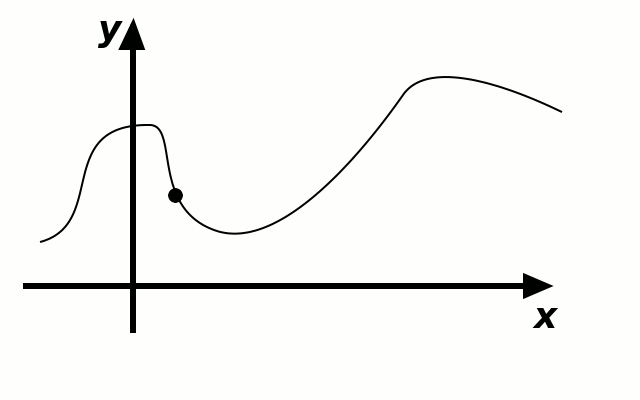# Derivatives

## Rate of Change

Consider the function $$f(x)=3x+7$$. We have that $$f(5)=3\cdot 5+7=22$$. The input value is $$5$$, and the output is $$22$$. If we increase the input by $$2$$, it becomes $$7$$ and the output becomes $$28$$. This means if the input increases by $$2$$, the output increases by $$6$$.

Assume now that the original input is increased by $$12$$. It becomes $$17$$. The output is $$f(17)=3\cdot 17+7=58$$, which is by $$36$$ bigger than the original output.

In general, if the input is increased by value $$t$$, the output increases by $$3\cdot t$$, and this number $$3$$ is called the rate of change.

We can study only the rate of change of a function. Let us consider the following example

Example 1 Consider the function $$f(x)=5x+1$$. Find the rate of change of $$f$$.

Now we may think that we are ready to define the rate of change for a general function. However, the following example is quite depressing. Consider the function $$g(x)=x^2$$.

Let’s start with $$x_O=3$$. We first find $$g(x_O)=9$$. If we take $$x_N=4$$, then $$g(x_N)=16$$ and the change in $$g$$ is $$\frac{16-9}{4-3}=7$$ times bigger than the change in $$x$$.

However, if we take $$x_{N^{\prime}}=5$$ we get $$g(x_{N^{\prime}})=25$$ and the change in $$g$$ is $$\frac{25-9}{5-3}=8$$ times bigger than the change in $$x$$.

If we take $$x_{N^{\prime\prime}}=500$$, then $$g(X_{N^{\prime\prime}})=250000$$ and the change in $$g$$ is $$\frac{250000-9}{500-3}=\frac{249999}{497}$$ times bigger than the change in $$x$$.

Despite this fact we are inclined to say that the rate of change of the function $$f(x)=x^2$$ is small. This is because nobody cares about the difference between $$g(500)$$ and $$g(3)$$.

We are interested in the infinitesimal rate of change, and this is called a derivative of the function.

## The definition

Definition of derivative

Assume that $$f$$ is a function, and $$a$$ a real number that belongs to the domain of definition of $$f$$. If the limit $$\lim_{h\to 0}\frac{f(a+h)-f(a)}h$$ exists, we say that the function $$f$$ is differentiable at point $$a$$ and we called the previous limit the derivative of $$f$$ at point $$a$$. We denote the derivative by $$f^{\prime}(a)$$, i.e.:

$f^{\prime}(a)=\lim_{h\to 0}\frac{f(a+h)-f(a)}h.$

## The slope of the tangentIf we draw the graph of the function $$f$$ in the $$xy$$ plane, then the slope of the line between the points $$(x,f(x))$$ and $$(x+\Delta x, f(x+\Delta x))$$ is equal to $$\frac{f(x+\Delta x)-f(x)}{\Delta x}$$.

As $$\Delta x$$ gets smaller and smaller, the line between $$(x,f(x))$$ and $$(x+\Delta x, f(x+\Delta x))$$ becomes a more accurate approximation to the tangent line of the graph of $$f$$. The quantity $\lim_{\Delta x\to0}\frac{f(x+\Delta x)-f(x)}{\Delta x)}$ corresponds to the slope of the tangent line to the graph of $$f$$ at the point $$(x,f(x))$$.

## Derivatives of $$x^m$$, $$e^x$$, $$\sin x$$, and $$\cos x$$

Our first theorem states that derivative of a constant function is $$0$$.

Theorem (Derivative of a constant)Let $$C$$ be arbitrary real number, and let $$f$$ be the function defined as $$f(x)=C$$ for all $$x\in\mathbb R$$. Then $$f$$ is differentiable for any $$a\in\mathbb R$$ and $$f^{\prime}(a)=0$$.

Our next theorem states that the derivative of a function of the form $$f(x)=x^m$$ is $$f^{\prime}(x)=mx^{m-1}$$ if $$m$$ is a positive integer.

Theorem (Power rule) Let $$m\in\mathbb N$$ and let $$f(x)=x^m$$. Then $$f^{\prime}(a)=ma^{m-1}$$ for all $$a\in\mathbb R$$.

The power rule holds even for $$m\in\mathbb R$$, but the proof is more complicated, and we will omit it for now.

Theorem (General power rule) Let $$m\in\mathbb R$$ and let $$f(x)=x^m$$. Then $$f^{\prime}(a)=ma^{m-1}$$ for all $$a\in\mathbb R$$.

Theorem (Derivative of the exponential function) If $$f(x)=e^x$$, then $$f^{\prime}(a)=e^a$$ for every real number $$a$$.

Theorem (Derivatives of $$\sin$$ and $$\cos$$) If $$f(x)=\sin x$$, then $$f^{\prime}(a)=-\cos a$$ for every real number $$a$$. If $$g(x)=\cos x$$, then $$g^{\prime}(a)=-\sin a$$.

## Practice Problems

Problem 1. Let $$g(x)=\cos x$$. Find $$g'(x)$$.

Problem 2. Let $$f(x)=x^3$$. Find $$f'(4)$$.

Problem 3. Let $$f(x)=x^3$$. Find $$f'(4)$$.

Problem 4 The definition of the derivative $$f^{\prime}(a)$$ of a function $$f$$ at a point $$a$$ states that $f^{\prime}(a)=\lim_{h\to0}\frac{f(a+h)-f(a)}h.$ This definition is equivalent to:
• (A) $$\displaystyle f^{\prime}(a)=\lim_{a\to 0}\frac{f(a+h)-f(a)}h$$
• (B) $$\displaystyle f^{\prime}(a)=\lim_{x\to a}\frac{f(a)-f(x)}x$$
• (C) $$\displaystyle f^{\prime}(a)=\lim_{x\to a}\frac{f(a)-f(x)}a$$
• (D) $$\displaystyle f^{\prime}(a)=\lim_{x\to a}\frac{f(a)-f(x)}{a-x}$$
• (E) $$\displaystyle f^{\prime}(a)=\lim_{x\to a}\frac{f(x)-f(a)}{a-x}$$

Problem 5. If $$f(x)=e^x$$, find $$f^{\prime}(e)$$.